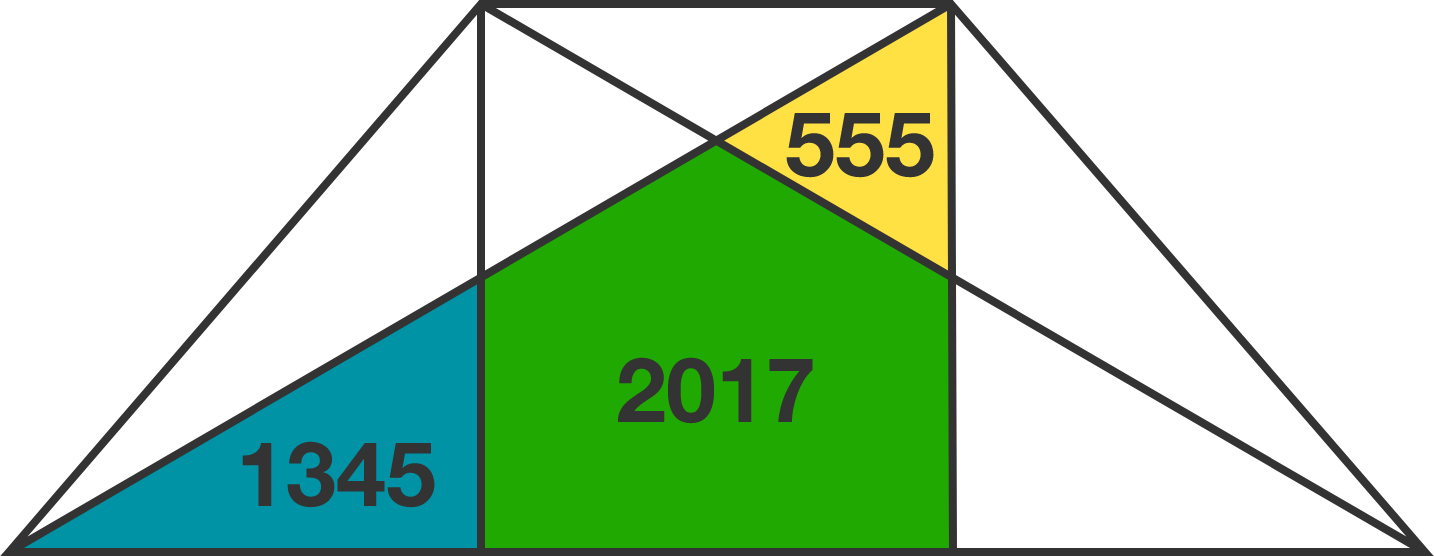# Symmetry does not clear everything

Geometry Level 2

As shown in the diagram below, inside a symmetrical quadrilateral, both its diagonals are drawn and two altitudes are dropped from the top vertices to the base. These segments divide the quadrilateral into eight parts, the areas of three of which are known to us: 555, 2017, 1345.

Find the area of the symmetrical quadrilateral.×